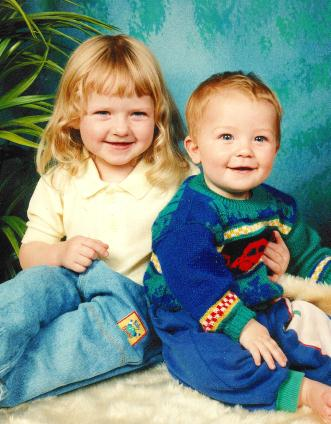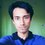# I Have Two Children...Do you like probability? Let's get down to business! Try your luck with the following problems!

"I have two children. The older of them is a girl. What is the probability that both of my children are girls?"

Seems simple enough, right? Since the older one is a girl, the younger one could either be a girl or a boy. Assuming that a boy and a girl both have an equal probability of being born, the answer should be $\frac{1}{2}$, right? Yes, you are correct. The answer is $\frac{1}{2}$.

Let's try a different version of this problem.

"I have two children. One of them is a girl. What is the probability that both of them are girls?"

Wait! How's this problem different from the last one? Well, for one the wording's a little different. But does that matter? If one of the children is a girl, the other one could either be a boy or a girl. So the probability should be $\frac{1}{2}$ like before, right?

Wrong!

While it is true that there are two possibilities for the other child, that doesn't mean that both of these events are equally probable. In this case, the event that the other child is a boy is twice more likely than the event that the other child is a girl. That is because it is twice more likely that the person has one boy and one girl than the event that the person has two girls. So the correct probability is $\frac{1}{3}$. There's a really good write-up on this here. That piece is so good that I advise you to stop reading this and start reading that. But don't forget to come back to this when you're done!

I'm sure that everything up to this point was pretty boring to a lot of people. Both of the problems above are very well-known. But now I'm going to take it up a notch. Let's go!

"I have two children. One of them is a girl who was born on a Friday. What is the probability that I have two girls?"

Wait a minute! How's this at all different from the second problem? What does Friday have to do with the probability?

Everything!

Do you remember the answer to the second problem? It was $\frac{1}{3}$. Adding the 'Friday condition' shifts the answer and the new answer is now closer to $\frac{1}{2}$ than it is to $\frac{1}{3}$!

Now this is the time I tell you stop reading and start thinking about the problem. It's a lot more fun than just reading the answer.

Congratulations to those who have resisted the urge to read on and gave the problem some thought!

So the answer is actually $\frac{13}{27}$. The solution itself isn't really that hard. There are two cases to consider.

$\text{Case 1}$: when the older child is a girl born on a Friday

There are $14$ different events for the younger child [a boy born on any day of the week + a girl born on any day of the week] and all of these are equally likely. We're only interested in the events where both the children are girls. There are $7$ of these.

$\text{Case 2}$: when the younger child is a girl born on a Friday

There are actually $13$ different events now [not $14$ since the event of (older sister born on a Friday + younger sister born on a Friday) was already counted in case $1$. We don't want to count that twice.]. And out of these we're interested in the ones where both of the children are girls. There are $6$ of these.

So, $\text{the probability that both of the children are girls}=\dfrac{7+6}{14+13}=\dfrac{13}{27}$

Simple, right?

If you're a little bit confused, don't worry. Take a piece of paper and a pen and go through the cases by yourself. You'll eventually figure it out.

The most interesting thing about this problem is that it's incredibly non-intuitive. Why on earth does the 'Friday condition' change the probability? Even if you don't say it loud, your children have to born on one day or another, right? So, why does saying it out loud change the probability? Well, because of the counter-intuitive nature of this problem, this note is going into my 'Counter-intuitive!' set. I'm going add some more stuff to it soon.

Until then!

Note: Throughout the entire note, we have disregarded the possibility of twins.

The picture was taken from here. It adds a nice touch to the post, don't you think? :)Note by Mursalin Habib
7 years ago

This discussion board is a place to discuss our Daily Challenges and the math and science related to those challenges. Explanations are more than just a solution — they should explain the steps and thinking strategies that you used to obtain the solution. Comments should further the discussion of math and science.

When posting on Brilliant:

• Use the emojis to react to an explanation, whether you're congratulating a job well done , or just really confused .
• Ask specific questions about the challenge or the steps in somebody's explanation. Well-posed questions can add a lot to the discussion, but posting "I don't understand!" doesn't help anyone.
• Try to contribute something new to the discussion, whether it is an extension, generalization or other idea related to the challenge.

MarkdownAppears as
*italics* or _italics_ italics
**bold** or __bold__ bold
- bulleted- list
• bulleted
• list
1. numbered2. list
1. numbered
2. list
Note: you must add a full line of space before and after lists for them to show up correctly
paragraph 1paragraph 2

paragraph 1

paragraph 2

[example link](https://brilliant.org)example link
> This is a quote
This is a quote
    # I indented these lines
# 4 spaces, and now they show
# up as a code block.

print "hello world"
# I indented these lines
# 4 spaces, and now they show
# up as a code block.

print "hello world"
MathAppears as
Remember to wrap math in $$ ... $$ or $ ... $ to ensure proper formatting.
2 \times 3 $2 \times 3$
2^{34} $2^{34}$
a_{i-1} $a_{i-1}$
\frac{2}{3} $\frac{2}{3}$
\sqrt{2} $\sqrt{2}$
\sum_{i=1}^3 $\sum_{i=1}^3$
\sin \theta $\sin \theta$
\boxed{123} $\boxed{123}$

Sort by:

Thanks for the informative post. As mentioned, a good way of interpreting this would be through the use of Venn diagrams. If I have time later today, I will add in how to visualize this "paradoxical" argument.

Staff - 7 years ago

When are you going to add Venn diagrams

- 2 years, 11 months ago

Another counter-intuitive scenario that I find very confusing is the Three Prisoners Problem.

- 7 years ago

Dude, you're giving away spoilers! I was going to cover this!

- 7 years ago

@Mursalin Habib Check my counter-intuitive problem- Birth Certificate! You may as well like to add it to your set...

- 7 years ago

Liked your problem and started following you! And i too like harry potter siries! Haha☺

- 7 years ago

Nice explanation!

- 7 years ago

I Am Not persuaded at all ....... I see that all cases must have the same Answer .. But You are good .. I am starting following you ... Hope you Best wishes ..

- 7 years ago

Here's something to get you thinking in the right direction for problem 2. Say you tossed two coins. Which is more likely? The event that you got two heads? Or the event that you got one head and one tail [not necessarily in that order]?

- 7 years ago

That is a great example! Mursalin Habib! I hope that the latter is 2/3?

- 7 years ago

The latter is actually $\frac{2}{4}$. Notice that we can't rule out the possibility of two tails.

If it were given that you had at least one head, then the answer would be $\frac{2}{3}$.

- 7 years ago

Correct. The point is, under which conditions may we apply which rules. May I try to confuse you?

Problem 1: "I have two children. The older of them is a girl. What is the probability that both of my children are girls?" = 1/2

Problem 2: "I have two children. One of them is a girl. What is the probability that both of them are girls?" = 1/3

Problem 3: "I have two children. One of them is a girl who was born on a Friday. What is the probability that I have two girls?" = 13/27

Consider problem 2 (you pose the question to me):

I know that you know on which specific weekday the girl was born. There are 7 possible cases. In each one of these 7 specific cases, according to problem 3, the answer is 13/27. Thus, the answer to problem 2 is 13/27 ...

Consider problem 2 again (you pose the question to me):

I know that you know the girl is either the older child or she is the younger one. In each one of these 2 possible cases, according to problem 1, the answer is 1/2. Thus, the answer to problem 2 is 1/2 ...

But the "official" answer to problem 2 is 1/3 ???

Eric Eerdekens

- 2 years, 9 months ago